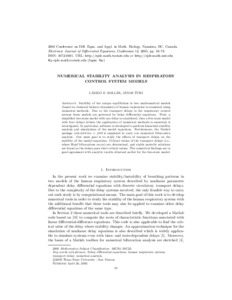Kollar, László E. and Turi, Janos (2005) Numerical Stability Analysis in Respiratory Control System Models. Electronic Journal of Differential Equations: Proceedings of 2004 Conference on Differential Equations and Applications in Mathematical Biology, 12. pp. 65-78.
Abstract

Stability of the unique equilibrium in two mathematical models (based on chemical balance dynamics) of human respiration is examined using numerical methods. Due to the transport delays in the respiratory control system these models are governed by delay differential equations. First, a simplified two-state model with one delay is considered, then a five-state model with four delays (where the application of numerical methods is essential) is investigated. In particular, software is developed to perform linearized stability analysis and simulations of the model equations. Furthermore, the Matlab package DDE-BIFTOOL v. 2.00 is employed to carry out numerical bifurcation analysis. Our main goal is to study the effects of transport delays on the stability of the model equations. Critical values of the transport delays (i.e., where Hopf bifurcations occur) are determined, and stable periodic solutions are found as the delays pass their critical values. The numerical findings are in good agreement with analytic results obtained earlier for the two-state model.

Information
Library
DocumentsPreview
kollar.pdf - Published Version